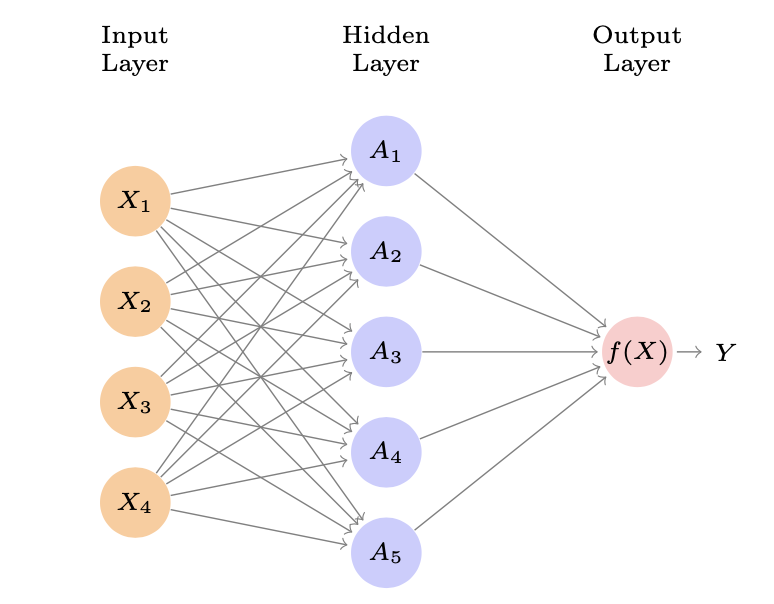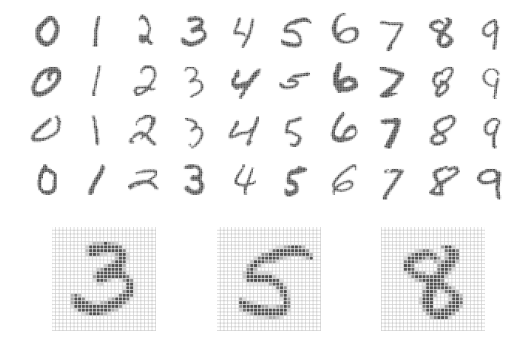## Deep Learning

• Neural networks became popular in the 1980s. Lots of successes, hype, and great conferences: NeurIPS, Snowbird

• Then along came SVMs, Random Forests and Boosting in the 1990s, and Neural Networks took a back seat

• Re-emerged around 2010 as Deep Learning. By 2020s very dominant and successful

• Part of success due to vast improvements in computing power, larger training sets, and software: Tensorflow and PyTorch

## Single Layer Neural Network

• $$f(x) = \beta_0 + \sum_{k=1}^{K} \beta_kh_k(X)$$

• Which equals: $$\beta_0 + \sum_{k=1}^{K} \beta_{k}g(w_{k0} + \sum_{j=1}^{p} w_{kj}X_j)$$• $$A_k = h_k(X) = g(w_{k0} + \sum_{j=1}^{p} w_{kj}X_j)$$ are called the activations in the hidden layer

• $$g(z)$$ is called the activation function. Popular are the sigmoid and rectified linear

• Activation functions in hidden layers are typically nonlinear, otherwise the model collapses to a linear model

• So the activations are like derived features — nonlinear transformations of linear combinations of the features

• The model is fit by minimizing $$\sum_{i=1}^{n} (y_i - f(x_i))^2$$ (for regression)

### Example: MNIST Digits

• Handwritten digits 28 × 28 grayscale images 60K train, 10K test images Features are the 784 pixel grayscale values $$\in$$ (0, 255) Labels are the digit class 0–9• Goal: build a classifier to predict the image class

• We build a two-layer network with 256 units at first layer, 128 units at second layer, and 10 units at output layer

• Along with intercepts (called biases) there are 235,146 parameters (referred to as weights)